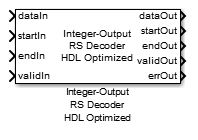# Integer-Output RS Decoder HDL Optimized

Decode data using Reed-Solomon (RS) decoder

• Library:
• Communications Toolbox HDL Support / Error Detection and Correction / Block

•## Description

The Integer-Output RS Decoder HDL Optimized block decodes data using RS decoder. The RS decoding follows the same standards as any other cyclic redundancy code. Use this block to model communications system forward error correction (FEC) codes. The block provides an architecture suitable for HDL code generation and hardware deployment.

For more information about the RS decoder, see the Integer-Output RS Decoder block. For more information on representing data for RS codes, see Integer Format (Reed-Solomon Only).

## Ports

### Input

expand all

Input data, specified as a scalar representing one symbol. For binary point scaling, the input data type must be an integer or `fixdt`. The `double` data type is allowed for simulation, but not for HDL code generation.

Data Types: `double` | `int8` | `int16` | `int32` | `int64` | `fixed point`

Start of input frame indicator, specified as a Boolean scalar.

Data Types: `Boolean`

End of input frame indicator, specified as a Boolean scalar.

Data Types: `Boolean`

Valid input data indicator, specified as a Boolean scalar.

This is a control signal that indicates if the data on the dataIn port is valid.

Data Types: `Boolean`

### Output

expand all

Decoded message data, returned as a scalar. This output data width is the same as the input data width.

Data Types: `double` | `int8` | `int16` | `int32` | `int64` | `fixed point`

Start of output frame indicator, returned as a Boolean scalar.

Data Types: `Boolean`

End of output frame indicator, returned as a Boolean scalar.

Data Types: `Boolean`

Valid output data indicator, returned as a Boolean scalar.

This is a control signal that indicates if the data on the dataOut port is valid.

Data Types: `Boolean`

Indications of corruption of the received data, returned as a Boolean scalar.

When this value is `1` (`true`) , the output contains at least one error. When this value is `0` (`false`), the output contains zero errors.

If the number of errors in the input codeword is greater than (Codeword lengthMessage length)/`2`, the block outputs data without correcting the errors and sets the errOut port to `1` (`true`) to indicate that errors that cannot be corrected exist in the input codeword.

Data Types: `Boolean`

Number of corrected errors, returned as a nonnegative scalar.

The maximum number of errors an RS code can correct is equal to (Codeword lengthMessage length)/2. If the number of errors in the input codeword is greater than (Codeword lengthMessage length)/2, the block outputs data without correcting the errors and sets the numErrors port to `0` to indicate that none of those errors can be corrected.

#### Dependencies

To enable this port, select the Output number of corrected symbol errors parameter.

Data Types: `uint8`

## Parameters

expand all

Specify the codeword length.

The codeword length `N` must be an integer equal to 2M – 1, where M is an integer in the range from 3 to 16. For more information on representing data for RS codes, see Integer Format (Reed-Solomon Only).

Specify the message length.

For more information on representing data for RS codes, see Integer Format (Reed-Solomon Only).

Specify the source of the primitive polynomial.

• Select `Auto` to specify the primitive polynomial based on the Codeword length parameter value. The degree of the primitive polynomial is calculated as M = `ceil`(log2(Codeword length). .

• Select `Property` to specify the primitive polynomial using the Primitive polynomial parameter.

Specify a binary row vector representing the primitive polynomial in descending order of powers.

For more information on how to specify a primitive polynomial, see Primitive Polynomials and Element Representations.

#### Dependencies

To enable this parameter, set the Source of primitive polynomial parameter to `Property`.

Specify the source of the starting power for roots of the primitive polynomial.

• Select `Property` to enable the B value parameter.

• Select `Auto`, to use the B value parameter default value of `1`.

The starting exponent of the roots.

#### Dependencies

To enable this parameter, set the Source of B, the starting power for roots of the primitive polynomial parameter to `Property`.

Select this parameter to enable the numErrors output port. This port outputs the number of corrected errors.

## Algorithms

expand all

This figure shows a sample output of the Integer-Output RS Decoder HDL Optimized block with a default configuration.## Version History

Introduced in R2012b